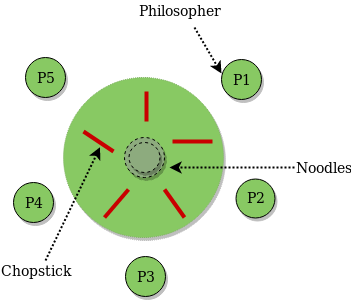# Dining Philosopher Problem Using Semaphores

The Dining Philosopher Problem – The Dining Philosopher Problem states that K philosophers seated around a circular table with one chopstick between each pair of philosophers. There is one chopstick between each philosopher. A philosopher may eat if he can pickup the two chopsticks adjacent to him. One chopstick may be picked up by any one of its adjacent followers but not both.Semaphore Solution to Dining Philosopher –

Each philosopher is represented by the following pseudocode:

```process P[i]
while true do
{  THINK;
PICKUP(CHOPSTICK[i], CHOPSTICK[i+1 mod 5]);
EAT;
PUTDOWN(CHOPSTICK[i], CHOPSTICK[i+1 mod 5])
}
```

There are three states of philosopher : THINKING, HUNGRY and EATING. Here there are two semaphores : Mutex and a semaphore array for the philosophers. Mutex is used such that no two philosophers may access the pickup or putdown at the same time. The array is used to control the behavior of each philosopher. But, semaphores can result in deadlock due to programming errors.

Code –

 `#include ` `#include ` `#include ` ` `  `#define N 5 ` `#define THINKING 2 ` `#define HUNGRY 1 ` `#define EATING 0 ` `#define LEFT (phnum + 4) % N ` `#define RIGHT (phnum + 1) % N ` ` `  `int` `state[N]; ` `int` `phil[N] = { 0, 1, 2, 3, 4 }; ` ` `  `sem_t mutex; ` `sem_t S[N]; ` ` `  `void` `test(``int` `phnum) ` `{ ` `    ``if` `(state[phnum] == HUNGRY ` `        ``&& state[LEFT] != EATING ` `        ``&& state[RIGHT] != EATING) { ` `        ``// state that eating ` `        ``state[phnum] = EATING; ` ` `  `        ``sleep(2); ` ` `  `        ``printf``(``"Philosopher %d takes fork %d and %d\n"``, ` `                      ``phnum + 1, LEFT + 1, phnum + 1); ` ` `  `        ``printf``(``"Philosopher %d is Eating\n"``, phnum + 1); ` ` `  `        ``// sem_post(&S[phnum]) has no effect ` `        ``// during takefork ` `        ``// used to wake up hungry philosophers ` `        ``// during putfork ` `        ``sem_post(&S[phnum]); ` `    ``} ` `} ` ` `  `// take up chopsticks ` `void` `take_fork(``int` `phnum) ` `{ ` ` `  `    ``sem_wait(&mutex); ` ` `  `    ``// state that hungry ` `    ``state[phnum] = HUNGRY; ` ` `  `    ``printf``(``"Philosopher %d is Hungry\n"``, phnum + 1); ` ` `  `    ``// eat if neighbours are not eating ` `    ``test(phnum); ` ` `  `    ``sem_post(&mutex); ` ` `  `    ``// if unable to eat wait to be signalled ` `    ``sem_wait(&S[phnum]); ` ` `  `    ``sleep(1); ` `} ` ` `  `// put down chopsticks ` `void` `put_fork(``int` `phnum) ` `{ ` ` `  `    ``sem_wait(&mutex); ` ` `  `    ``// state that thinking ` `    ``state[phnum] = THINKING; ` ` `  `    ``printf``(``"Philosopher %d putting fork %d and %d down\n"``, ` `           ``phnum + 1, LEFT + 1, phnum + 1); ` `    ``printf``(``"Philosopher %d is thinking\n"``, phnum + 1); ` ` `  `    ``test(LEFT); ` `    ``test(RIGHT); ` ` `  `    ``sem_post(&mutex); ` `} ` ` `  `void``* philospher(``void``* num) ` `{ ` ` `  `    ``while` `(1) { ` ` `  `        ``int``* i = num; ` ` `  `        ``sleep(1); ` ` `  `        ``take_fork(*i); ` ` `  `        ``sleep(0); ` ` `  `        ``put_fork(*i); ` `    ``} ` `} ` ` `  `int` `main() ` `{ ` ` `  `    ``int` `i; ` `    ``pthread_t thread_id[N]; ` ` `  `    ``// initialize the semaphores ` `    ``sem_init(&mutex, 0, 1); ` ` `  `    ``for` `(i = 0; i < N; i++) ` ` `  `        ``sem_init(&S[i], 0, 0); ` ` `  `    ``for` `(i = 0; i < N; i++) { ` ` `  `        ``// create philosopher processes ` `        ``pthread_create(&thread_id[i], NULL, ` `                       ``philospher, &phil[i]); ` ` `  `        ``printf``(``"Philosopher %d is thinking\n"``, i + 1); ` `    ``} ` ` `  `    ``for` `(i = 0; i < N; i++) ` ` `  `        ``pthread_join(thread_id[i], NULL); ` `} `

Note – The below program may compile only with C compilers with semaphore and pthread library.

Attention reader! Don’t stop learning now. Get hold of all the important CS Theory concepts for SDE interviews with the CS Theory Course at a student-friendly price and become industry ready.

My Personal Notes arrow_drop_upCheck out this Author's contributed articles.

If you like GeeksforGeeks and would like to contribute, you can also write an article using contribute.geeksforgeeks.org or mail your article to contribute@geeksforgeeks.org. See your article appearing on the GeeksforGeeks main page and help other Geeks.

Please Improve this article if you find anything incorrect by clicking on the "Improve Article" button below.

Improved By : ssatyanand7

Article Tags :
Practice Tags :

5

Please write to us at contribute@geeksforgeeks.org to report any issue with the above content.